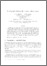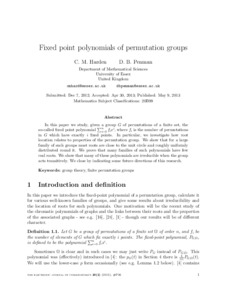# Fixed Point Polynomials of Permutation Groups

Harden, CM and Penman, DB (2013) 'Fixed Point Polynomials of Permutation Groups.' The Electronic Journal of Combinatorics, 20 (2). ISSN 1077-8926Preview
Text
cmhpaper.pdf

<jats:p>In this paper we study, given a group $G$ of permutations of a finite set, the so-called fixed point polynomial $\sum_{i=0}^{n}f_{i}x^{i}$, where $f_{i}$ is the number of permutations in $G$ which have exactly $i$ fixed points. In particular, we investigate how root location relates to properties of the permutation group. We show that for a large family of such groups most roots are close to the unit circle and roughly uniformly distributed round it. We prove that many families of such polynomials have few real roots. We show that many of these polynomials are irreducible when the group acts transitively. We close by indicating some future directions of this research. A corrigendum was appended to this paper on 10th October 2014. </jats:p>View Item